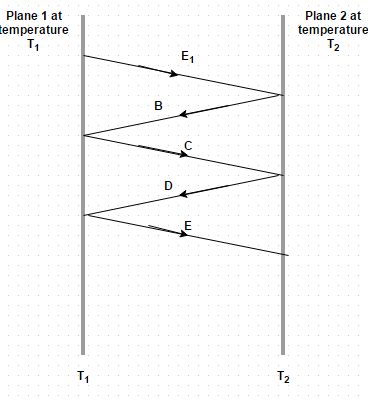# Test: Heat Exchange Between Non Black Bodies

## 10 Questions MCQ Test Heat Transfer for Engg. | Test: Heat Exchange Between Non Black Bodies

Description
This mock test of Test: Heat Exchange Between Non Black Bodies for Chemical Engineering helps you for every Chemical Engineering entrance exam. This contains 10 Multiple Choice Questions for Chemical Engineering Test: Heat Exchange Between Non Black Bodies (mcq) to study with solutions a complete question bank. The solved questions answers in this Test: Heat Exchange Between Non Black Bodies quiz give you a good mix of easy questions and tough questions. Chemical Engineering students definitely take this Test: Heat Exchange Between Non Black Bodies exercise for a better result in the exam. You can find other Test: Heat Exchange Between Non Black Bodies extra questions, long questions & short questions for Chemical Engineering on EduRev as well by searching above.
QUESTION: 1

### Consider radiant heat exchange between two non-black parallel surfaces. The surface 1 emits radiant energy E 1 which strikes the surface 2. Identify the correct optionSolution:

The surface 1 emits radiant energy E 1 which strikes the surface 2. From it a part α2 is absorbed by the surface 2 and the remainder (1 – α2) E 1 is reflected back to surface 1 and so on.

QUESTION: 2

### A large plane, perfectly insulated on one face and maintained at a fixed temperature T 1 on the bare face, has an emissivity of 0.84 and loses 250 W/m2 when exposed to surroundings at nearly 0 K. The radiant heat loss from another plane of the same size is 125 W/m2 when bare face having emissivity 0.42 and is maintained at temperature T 2 is exposed to the same surroundings. Subsequently these two planes are brought together so that the parallel bare faces lie only 1 cm apart and the heat supply to each is so regulated that their respective temperatures T 1 and T 2 remains unchanged. Determine he net heat flux between the planes

Solution:

12 = F 12 σ b (T 14 – T 24). Since T 1 = T 2, we get Q 12/A1 = 0.

QUESTION: 3

### Interchange factor for body 1 completely enclosed by body 2 (body 1 is large) is given by

Solution:

This is the interchange factor for the radiation from surface 1 to surface 2.

QUESTION: 4

A thermos flask has a double walled bottle and the space between the walls is evacuated so as to reduce the heat flow. The bottle surfaces are silver plated and the emissivity of each surface is 0.025. If the contents of the bottle are at 375 K, find the rate of heat loss from the thermos bottle to the ambient air at 300 K

Solution:

12 = F 12 σ b (T 14 – T 24). F 12 = 1/ (1/E 1 + 1/E– 1) = 0.01266.

QUESTION: 5

A 250 mm by 250 mm ingot casting, 1.5 m high and at 1225 K temperature, is stripped from its mold. The casting is made to stand on end on the floor of a large foundry whose wall, floor and roof can be assumed to be at 300 K temperature. Make calculation for the rate of radiant heat interchange between the casting and the room. The casting material has an emissivity of 0.85

Solution:

12 = F 12 σ b (T 14 – T 24). F 12 = 0.85 and A 1 = (0.25)2 + 4(1.5) (0.25) = 1.5625 m2.

QUESTION: 6

Interchange factor for infinitely long concentric cylinders is given by

Solution:

This is the interchange factor for the radiation from surface 1 to surface 2.

QUESTION: 7

What is the geometric factor for infinitely long concentric cylinders?

Solution:

The inner cylinder is completely enclosed by the outer cylinder and as such the entire heat radiations emitted by the emitted by the inner cylinder are intercepted by the outer cylinder.

QUESTION: 8

What is the geometric factor for concentric spheres?

Solution:

The inner sphere is completely enclosed by the outer sphere and as such the entire heat radiations emitted by the emitted by the inner sphere are intercepted by the outer cylinder.

QUESTION: 9

The net heat interchange between non-black bodies at temperature T1 and Tis given by

Solution:

The factor f12 is called the interchanging factor from surface 1 to surface 2.

QUESTION: 10

A thermos flask has a double walled bottle and the space between the walls is evacuated so as to reduce the heat flow. The bottle surfaces are silver plated and the emissivity of each surface is 0.025. If the contents of the bottle are at 375 K and temperature of ambient air is 300 K. What thickness of cork (k = 0.03 W/m degree) would be required if the same insulating effect is to be achieved by the use of cork?

Solution:

Q = k A (t 1 – t 2)/δ. So, δ = 0.268 m = 26.8 cm.